##### Geometry Essentials For Dummies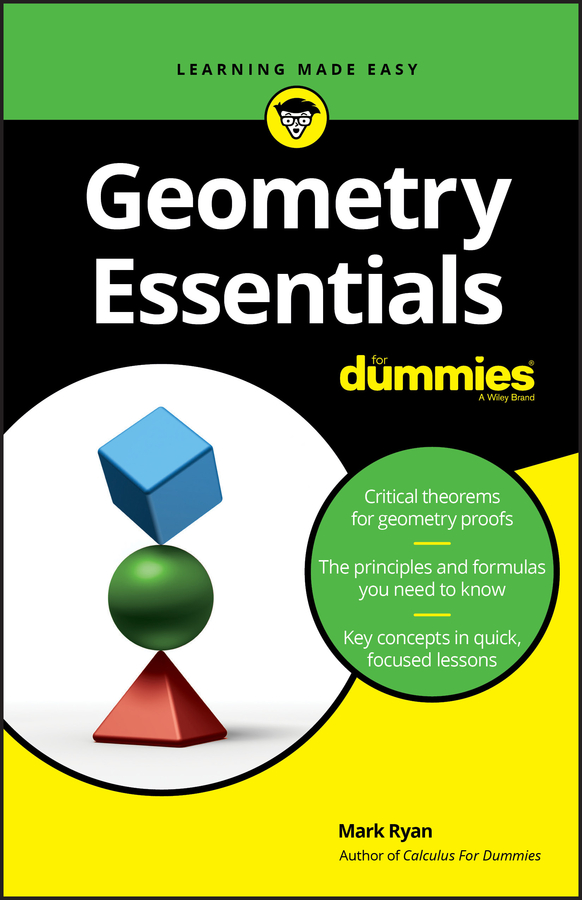To find the number of diagonals in a polygon with n sides, use the following formula: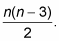This formula looks like it came outta nowhere, doesn’t it? Of course, no math formulas come out of nowhere, but you might have to think about this one a bit to discover the logic behind it. (Just memorizing it is okay, but what’s the fun in that?)

Here’s where the diagonal formula comes from and why it works. Each diagonal connects one point to another point in the polygon that isn’t its next-door neighbor. In an n-sided polygon, you have n starting points for diagonals. And each diagonal can go to (n – 3) ending points because a diagonal can’t end at its own starting point or at either of the two neighboring points. So the first step is to multiply n by (n – 3). Then, because each diagonal’s ending point can be used as a starting point as well, the product n(n – 3) counts each diagonal twice. That’s why you divide by 2.

Here’s a problem for you: If a polygon has 90 diagonals, how many sides does it have?

You know what the formula for the number of diagonals in a polygon is, and you know that the polygon has 90 diagonals, so plug 90 in for the answer and solve for n: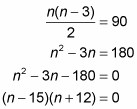Thus, n equals 15 or –12. But because a polygon can’t have a negative number of sides, n must be 15. So you have a 15-sided polygon (a pentadecagon, in case you’re curious).

Here’s a nifty real-world application of the diagonal formula. Say there’s a small tennis tournament with six people in which everyone has to play everyone else. How many total matches will there be? The following figure shows the six tennis players with segments connecting each pair of players.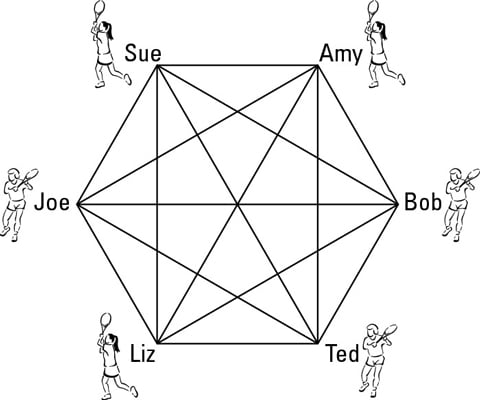Each segment represents a match between two contestants. So to get the total number of matches, you just have to count up all the segments in the figure: the number of sides of the hexagon (6) plus the number of diagonals in the hexagon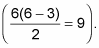The total is therefore 15 matches. For the general case, the total number of matches in a round-robin tournament with n players would be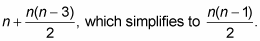Game, set, match.